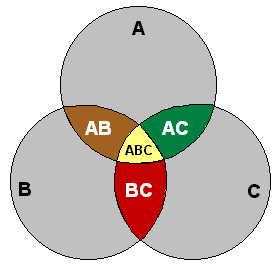# Venn diagram

(redirected from Ven diagram)
Also found in: Dictionary, Thesaurus, Medical.

## Venn diagram

Maths logic a diagram in which mathematical sets or terms of a categorial statement are represented by overlapping circles within a boundary representing the universal set, so that all possible combinations of the relevant properties are represented by the various distinct areas in the diagram

## Venn diagram

[′ven ‚dī·ə‚gram]
(mathematics)
A pictorial representation of set theoretic operations such as union, intersection, and complementation of sets.

## Venn diagram

A graphic technique for visualizing set theory concepts using overlapping circles and shading to indicate intersection, union and complement. It was introduced in the late 1800s by English logician, John Venn, although it is believed that the method originated earlier.

A Venn DiagramVenn diagrams show how items relate to each other. Most of the "action" is in the overlapping areas.
Site: Follow: Share:
Open / Close